# Generate Realistic Human Face using GAN

This article contain a brief intro to Generative Adversarial Network(GAN) and how to build a Human Face Generator.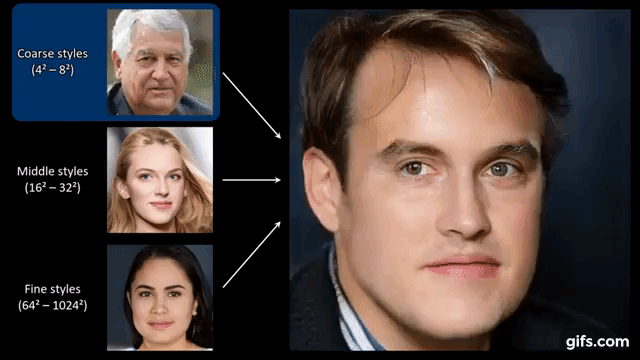“The coolest idea in deep learning in the last 20 years.” — Yann LeCun on GANs.

We heard the news on Artistic Style Transfer and face-swapping applications  (aka deepfakes), Natural Voice Generation (Google Duplex), Music Synthesissmart replysmart compose, etc.

The technology behind these kinds of AI is called a GAN, or “Generative Adversarial Network”. A GAN takes a different approach to learning than other types of neural networks. GANs algorithmic architectures that use two neural networks called a Generator and a Discriminator, which “compete” against one another to create the desired result. The Generator’s job is to create realistic-looking fake images, while the Discriminator’s job is to distinguish between real images and fake images. If both are functioning at high levels, the result is images that are seemingly identical real-life photos.

Generative Adversarial Networks have had a huge success since they were introduced in 2014 by Ian J. Goodfellow and co-authors in the article Generative Adversarial Nets.

### Why were GANs developed in the first place?

It has been noticed most of the mainstream neural nets can be easily fooled into misclassifying things by adding only a small amount of noise into the original data. Surprisingly, the model after adding noise has higher confidence in the wrong prediction than when it predicted correctly. The reason for such an adversary is that most machine learning models learn from a limited amount of data, which is a huge drawback, as it is prone to overfitting. Also, the mapping between the input and the output is almost linear. Although it may seem that the boundaries of separation between the various classes are linear, in reality, they are composed of linearities and even a small change in a point in the feature space might lead to misclassification of data.### How does GANs work?

GANs learn a probability distribution of a dataset by pitting two neural networks against each other.

One neural network, called the Generator, generates new data instances, while the other, the Discriminator, evaluates them for authenticity; i.e. the discriminator decides whether each instance of data that it reviews belongs to the actual training dataset or not.

Meanwhile, the generator is creating new, synthetic/fake images that it passes to the discriminator. It does so in the hopes that they, too, will be deemed authentic, even though they are fake. The fake image is generated from a 100-dimensional noise (uniform distribution between -1.0 to 1.0) using the inverse of convolution, called transposed convolution.

The goal of the generator is to generate passable images: to lie without being caught. The goal of the discriminator is to identify images coming from the generator as fake.

Here are the steps a GAN takes:

• The generator takes in random numbers and returns an image.
• This generated image is fed into the discriminator alongside a stream of images taken from the actual, ground-truth dataset.
• The discriminator takes in both real and fake images and returns probabilities, a number between 0 and 1, with 1 representing a prediction of authenticity and 0 representing fake.

So you have a double feedback loop:

• The discriminator is in a feedback loop with the ground truth of the images, which we know.
• The generator is in a feedback loop with the discriminator.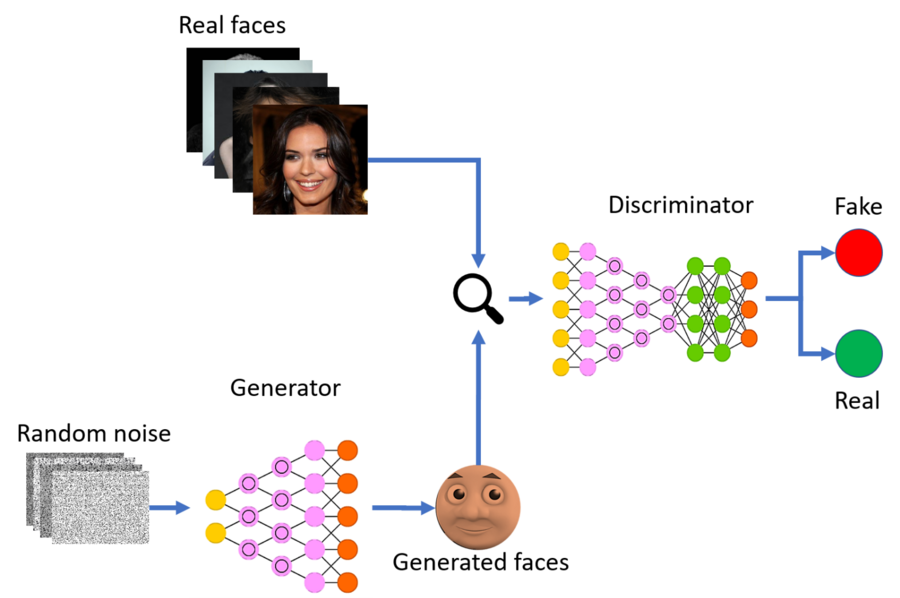### The math behind the GANs

Let’s dig a little deeper and understand how it works mathematically. Discriminator’s job is to perform Binary Classification to detect between Real and Fake so its loss function is Binary Cross Entropy. What Generator does is Density Estimation, from the noise to real data, and feed it to Discriminator to fool it.

The approach followed in the design is to model it as a MiniMax game. Now let’s have a look at cost functions: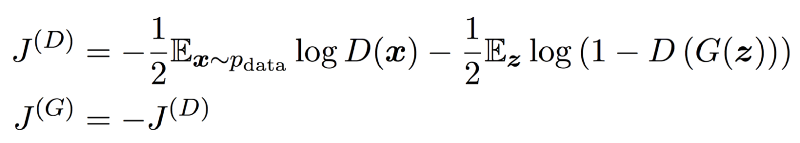The first term in `J(D)` represents feeding the actual data to the discriminator, and the discriminator would want to maximize the log probability of predicting one, indicating that the data is real. The second term represents the samples generated by `G`. Here, the discriminator would want to maximize the log probability of predicting zero, indicating the data is fake. The generator, on the other hand, tries to minimize the log probability of the discriminator being correct. The solution to this problem is an equilibrium point of the game, which is a saddle point of the discriminator loss.

Objective Function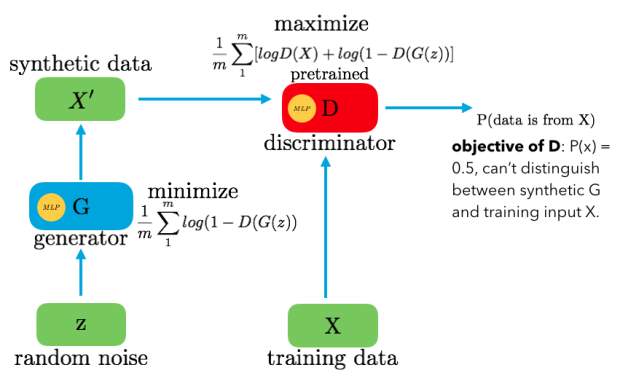Architecture of GANs

`D()` gives us the probability that the given sample is from training data X. For the Generator, we want to minimize `log(1-D(G(z))` i.e. when the value `D(G(z))` is high then `D` will assume that `G(z)` is nothing but X and this makes `1-D(G(z))` very low and we want to minimize it which this even lower. For the Discriminator, we want to maximize `D(X)` and `(1-D(G(z)))`. So the optimal state of D will be `P(x)=0.5`. However, we want to train the generator G such that it will produce the results for the discriminator D so that D won’t be able to distinguish between z and X.

Now the question is why this is a minimax function? It is because the Discriminator tries to maximize the objective while the Generator tries to minimize it, due to this minimizing/maximizing we get the minimax term. They both learn together by alternating gradient descent.

While the idea of GAN is simple in theory, it is very difficult to build a model that works. In GAN, there are two deep networks coupled together making backpropagation of gradients twice as challenging.

Deep Convolutional GAN (DCGAN) is one of the models that demonstrated how to build a practical GAN that can learn by itself how to synthesize new images. DCGAN is very similar to GANs but specifically focuses on using deep convolutional networks in place of fully-connected networks used in Vanilla GANs.

Convolutional networks help in finding deep correlation within an image, that is they look for spatial correlation. This means DCGAN would be a better option for image/video data, whereas GANs can be considered as a general idea on which DCGAN and many other architectures (CGAN, CycleGAN, StarGAN and many others) have been developed.

### Dataset

This dataset is great for training and testing models for face detection, particularly for recognizing facial attributes such as finding people with brown hair, are smiling, or wearing glasses. Images cover large pose variations, background clutter, diverse people, supported by a large number of images and rich annotations.

The dataset can be downloaded from Kaggle. Our objective is to create a model capable of generating realistic human images that do not exist in reality.

You heard it right!

Let us load the dataset and see how the input images look like:

``````
from tqdm import tqdm
import numpy as np
import pandas as pd
import os
from matplotlib import pyplot as plt
PIC_DIR = './drive/img_align_celeba/'
IMAGES_COUNT = 10000
ORIG_WIDTH = 178
ORIG_HEIGHT = 208
diff = (ORIG_HEIGHT - ORIG_WIDTH) // 2
WIDTH = 128
HEIGHT = 128
crop_rect = (0, diff, ORIG_WIDTH, ORIG_HEIGHT - diff)
images = []
for pic_file in tqdm(os.listdir(PIC_DIR)[:IMAGES_COUNT]):
pic = Image.open(PIC_DIR + pic_file).crop(crop_rect)
pic.thumbnail((WIDTH, HEIGHT), Image.ANTIALIAS)
images.append(np.uint8(pic)) #Normalize the images
images = np.array(images) / 255
images.shape #print first 25 images
plt.figure(1, figsize=(10, 10))
for i in range(25):
plt.subplot(5, 5, i+1)
plt.imshow(images[i])
plt.axis('off')
plt.show()
``````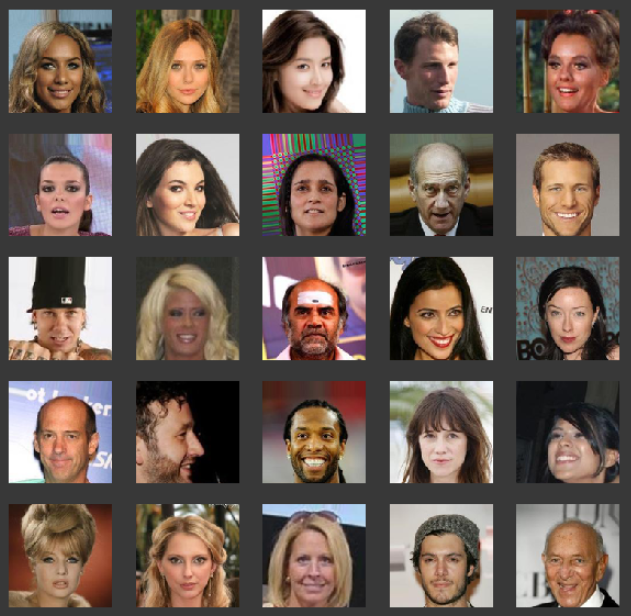Input images

Next step is to create a Generator:

The generator goes the other way: It is the artist who is trying to fool the discriminator. This network consists of 8 convolutional layers. Here first, we take our input, called `gen_input` and feed it into our first convolutional layer. Each convolutional layer performs a convolution and then performs batch normalization and a leaky ReLu as well. Then, we return the tanh activation function.

``````
LATENT_DIM = 32
CHANNELS = 3
def create_generator():
gen_input = Input(shape=(LATENT_DIM, ))

x = Dense(128 * 16 * 16)(gen_input)
x = LeakyReLU()(x)
x = Reshape((16, 16, 128))(x)

x = LeakyReLU()(x)

x = Conv2DTranspose(256, 4, strides=2, padding='same')(x)
x = LeakyReLU()(x)

x = Conv2DTranspose(256, 4, strides=2, padding='same')(x)
x = LeakyReLU()(x)

x = Conv2DTranspose(256, 4, strides=2, padding='same')(x)
x = LeakyReLU()(x)

x = LeakyReLU()(x)
x = LeakyReLU()(x)
x = Conv2D(CHANNELS, 7, activation='tanh', padding='same')(x)

generator = Model(gen_input, x)
return generator
``````

Next, create a Discriminator:

The discriminator network consists of convolutional layers the same as the generator. For every layer of the network, we are going to perform a convolution, then we are going to perform batch normalization to make the network faster and more accurate and finally, we are going to perform a Leaky ReLu.

``````
def create_discriminator():
disc_input = Input(shape=(HEIGHT, WIDTH, CHANNELS))

x = Conv2D(256, 3)(disc_input)
x = LeakyReLU()(x)

x = Conv2D(256, 4, strides=2)(x)
x = LeakyReLU()(x)

x = Conv2D(256, 4, strides=2)(x)
x = LeakyReLU()(x)

x = Conv2D(256, 4, strides=2)(x)
x = LeakyReLU()(x)

x = Conv2D(256, 4, strides=2)(x)
x = LeakyReLU()(x)

x = Flatten()(x)
x = Dropout(0.4)(x)

x = Dense(1, activation='sigmoid')(x)
discriminator = Model(disc_input, x)

optimizer = RMSprop(
lr=.0001,
clipvalue=1.0,
decay=1e-8
)

discriminator.compile(
optimizer=optimizer,
loss='binary_crossentropy'
)

return discriminator
``````

Define a GAN Model:

Next, a GAN model can be defined that combines both the generator model and the discriminator model into one larger model. This larger model will be used to train the model weights in the generator, using the output and error calculated by the discriminator model. The discriminator model is trained separately, and as such, the model weights are marked as not trainable in this larger GAN model to ensure that only the weights of the generator model are updated. This change to the trainability of the discriminator weights only affects when training the combined GAN model, not when training the discriminator standalone.

This larger GAN model takes as input a point in the latent space, uses the generator model to generate an image, which is fed as input to the discriminator model, then output or classified as real or fake.

Since the output of the Discriminator is sigmoid, we use binary cross-entropy for the loss. RMSProp as an optimizer generates more realistic fake images compared to Adam for this case. The learning rate is 0.0001. Weight decay and clip value stabilize learning during the latter part of the training. You have to adjust the decay if you want to adjust the learning rate.

GANs try to replicate a probability distribution. Therefore, we should use loss functions that reflect the distance between the distribution of the data generated by the GAN and the distribution of the real data.

``````
generator = create_generator()
discriminator = create_discriminator()
discriminator.trainable = False
gan_input = Input(shape=(LATENT_DIM, ))
gan_output = discriminator(generator(gan_input))
optimizer = RMSprop(lr=.0001, clipvalue=1.0, decay=1e-8)
gan.compile(optimizer=optimizer, loss='binary_crossentropy')
``````

Rather than just having a single loss function, we need to define three: The loss of the generator, the loss of the discriminator when using real images and the loss of the discriminator when using fake images. The sum of the fake image and real image loss is the overall discriminator loss.

Training the GAN model:

Training is the hardest part and since a GAN contains two separately trained networks, its training algorithm must address two complications:

• GANs must juggle two different kinds of training (generator and discriminator).
• GAN convergence is hard to identify.

As the generator improves with training, the discriminator performance gets worse because the discriminator can’t easily tell the difference between real and fake. If the generator succeeds perfectly, then the discriminator has a 50% accuracy. In effect, the discriminator flips a coin to make its prediction.

This progression poses a problem for convergence of the GAN as a whole: the discriminator feedback gets less meaningful over time. If the GAN continues training past the point when the discriminator is giving completely random feedback, then the generator starts to train on junk feedback, and its quality may collapse.

``````
iters = 20000
batch_size = 16RES_DIR = 'res2'
FILE_PATH = '%s/generated_%d.png'
if not os.path.isdir(RES_DIR):
os.mkdir(RES_DIR)
CONTROL_SIZE_SQRT = 6
control_vectors = np.random.normal(size=(CONTROL_SIZE_SQRT**2, LATENT_DIM)) / 2
start = 0
d_losses = []
a_losses = []
images_saved = 0
for step in range(iters):
start_time = time.time()
latent_vectors = np.random.normal(size=(batch_size, LATENT_DIM))
generated = generator.predict(latent_vectors)

real = images[start:start + batch_size]
combined_images = np.concatenate([generated, real])

labels = np.concatenate([np.ones((batch_size, 1)), np.zeros((batch_size, 1))])
labels += .05 * np.random.random(labels.shape)

d_loss = discriminator.train_on_batch(combined_images, labels)
d_losses.append(d_loss)

latent_vectors = np.random.normal(size=(batch_size, LATENT_DIM))

a_losses.append(a_loss)

start += batch_size
if start > images.shape - batch_size:
start = 0

if step % 50 == 49:
gan.save_weights('gan.h5')

print('%d/%d: d_loss: %.4f,  a_loss: %.4f.  (%.1f sec)' % (step + 1, iters, d_loss, a_loss, time.time() - start_time))

control_image = np.zeros((WIDTH * CONTROL_SIZE_SQRT, HEIGHT * CONTROL_SIZE_SQRT, CHANNELS))
control_generated = generator.predict(control_vectors)
for i in range(CONTROL_SIZE_SQRT ** 2):
x_off = i % CONTROL_SIZE_SQRT
y_off = i // CONTROL_SIZE_SQRT
control_image[x_off * WIDTH:(x_off + 1) * WIDTH, y_off * HEIGHT:(y_off + 1) * HEIGHT, :] = control_generated[i, :, :, :]
im = Image.fromarray(np.uint8(control_image * 255))
im.save(FILE_PATH % (RES_DIR, images_saved))
images_saved += 1
``````

Let us also make the GIF of the output images that have been generated.

``````
import imageio
import shutil
images_to_gif = []
for filename in os.listdir(RES_DIR):
imageio.mimsave('trainnig_visual.gif', images_to_gif)
shutil.rmtree(RES_DIR)
``````

Output

You can get the code in my GitHub repo.

nageshsinghc4/Face-generation-GAN

We just saw how a model can generate almost a human-like face if trained sufficiently. Due to computation constraints, I have trained the model for 15000 epochs. You can try with more epochs to get even better results.

“GANs are Dangerous”

As time goes on, these algorithms that exist all around us get better and better at what they do, meaning these generative models will likely get better at generating imitative objects. It is highly likely that another groundbreaking generative model is just on the horizon.

This technology can be used for many good things. However, the potential for bad is there as well. Recall the 2016 election and many subsequent international elections, where false news articles flooded almost all social media platforms. Imagine the impact these articles would have had if they had contained accompanying “false images” and “false audio”. Propaganda would likely spread far more easily in such a world. Essentially, these new generative models, with enough time and data, they can generate very convincing samples from almost any distribution.

You can go to thispersondoesnotexist.com and can feel the power of GAN models, every time you refresh the website you will see a different human figure which doesn't even exist and has been generated via GAN. It's truly fascinating.

### Conclusion: The Future of GANs

Unsupervised learning is the next frontier in artificial intelligence and we are moving towards it.

GANs and generative models general are very fun and perplexing. They encapsulate another step towards a world where we depend more and more on artificial intelligence. GANs have a huge number of applications in cases such as Generating examples for Image Datasets, Generating Realistic Photographs, Image-to-Image Translation, Text-to-Image Translation, Semantic-Image-to-Photo Translation, Face Frontal View Generation, Generate New Human Poses, Face Aging, Video Prediction, 3D Object Generation, etc.

Well, this concludes this article on GANs where we have discussed this cool domain of AI and how it is practically implemented. I hope you guys have enjoyed reading it, feel free to share your comments/thoughts/feedback in the comment section.